## Distance

Let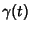be a smooth curve in a Manifoldfromtowithand. Then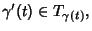where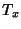is the Tangent Space ofat. The Length of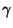with respect to the Riemannian structure is given by(1)

and the distancebetweenandis the shortest distance betweenandgiven by(2)

In order to specify the relative distances ofpoints in the plane,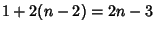coordinates are needed, since the first can always be taken as (0, 0) and the second as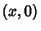, which defines the x-Axis. The remaining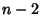points need two coordinates each. However, the total number of distances is(3)

where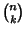is a Binomial Coefficient. The distances betweenpoints are therefore subject torelationships, where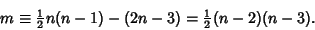(4)

For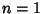, 2, ..., this gives 0, 0, 0, 1, 3, 6, 10, 15, 21, 28, ... (Sloane's A000217) relationships, and the number of relationships betweenpoints is the Triangular Number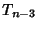.

Although there are no relationships for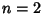and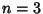points, for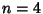(a Quadrilateral), there is one (Weinberg 1972):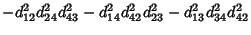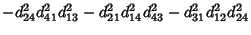(5)

This equation can be derived by writing(6)

and eliminating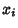and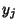from the equations for,,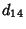,,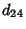, and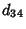.

See also Arc Length, Cube Point Picking, Expansive, Length (Curve), Metric, Planar Distance, Point-Line Distance--2-D, Point-Line Distance--3-D, Point-Plane Distance, Point-Point Distance--1-D, Point-Point Distance--2-D, Point-Point Distance--3-D, Space Distance, Sphere

References

Gray, A. The Intuitive Idea of Distance on a Surface.'' §13.1 in Modern Differential Geometry of Curves and Surfaces. Boca Raton, FL: CRC Press, pp. 251-255, 1993.

Sloane, N. J. A. Sequence A000217/M2535 in An On-Line Version of the Encyclopedia of Integer Sequences.'' http://www.research.att.com/~njas/sequences/eisonline.html and Sloane, N. J. A. and Plouffe, S. The Encyclopedia of Integer Sequences. San Diego: Academic Press, 1995.

Weinberg, S. Gravitation and Cosmology: Principles and Applications of the General Theory of Relativity. New York: Wiley, p. 7, 1972.